Advanced Introduction to C++, Scientific Computing and Machine Learning

Claudius Gros, WS 2021/22

Institut für theoretische Physik
Goethe-University Frankfurt a.M.

simple vs. complex problemssimple problems

• limited number of variables
• exact solution of interest, like for XOR
: lowest energy state
: gradient descent does not work
backpropagation fails for simple problems

complex problems

• large numbers of variables
• many 'good' local minima are ok
• humans would not survive, if it was otherwise
given enough (labeled) training data, large
classes of complex problems are 'solvable'
• like playing Go, recognizing a face, ...
• counter-examples: cryptology, ...

deep networkspruning
 removing : weak links; $|w_{ij}|$ well below average reduces : network complexity; overfitting

data preprocessing
 whitening : covariance matrix $\to$ identity matrix : all data equally relevant
• a large number of hidden layes
allows for highly non-linear and
hierarchical classification
• huge number of parameters and options,
for training and networks design
• learing rate $\to$ adaptive control
• initialization of weights
• ...
• 'shallow nets' have not too many hidden layers

batch learning

• synaptic weights adjusted after presenting a 'batch'
of $N_d\$ training data $\ \ (\mathbf{I}_\alpha,\mathbf{y}_\alpha)$

$\displaystyle E = \frac{1}{2 N_d } \sum_\alpha \big|\mathbf{y}_\alpha-\mathbf{y}\big|^2, \qquad\quad \mathbf{y}=\mathbf{y}(\mathbf{I}_\alpha)$

'online' learning

• ML
:training on a pair by pair basis
• neurosciences
: if the cognitive system is continuously active
: no distinct phases for learing and performing

offline learning

• distinct modes for trainging and predicting
: most ML algorithms are offline (but not the brain)

deep belief nets (DBN)stacked RBMs

• data on input layer
train
input layer $\ \leftrightarrow\$ first hidden layer
• first hidden layer acts as data
train
first hidden layer $\ \leftrightarrow\$ second hidden layer
• repeat
 $\to$ hierarchical feature representation by hidden nodes

data availability

• large amount of unlabelled data
images from web, ...
• much smaller amount of labelled data

semi-supervised learning

• unsupervised pre-training followed by supervised learning
train a net of stacked RBMs with unlabelled data
add a final output node connected to top hidden layer
use backpropagation on labelled data
to fine-tune connection weights
• graph undirected, besides links to output layer

autoencoderdimensionality reduction

$\fbox{$\phantom{\big|}$input$\phantom{\big|}$} \quad\equiv\quad \fbox{$\phantom{\big|}$output$\phantom{\big|}$}$

• hidden layer with low numbers of units
$\to\$ information bottleneck
autoencoders generate low-dimensional
representations of the data
• training via backpropagation of errors

denoising

• using on-purpose corrupted input data
forces the autencoder then learns to
reconstruct the orginal data

stacked autoencoders

• two meanings
• several hidden layers
• stacked to form a deep network
• stacking for deep networks
: bottleneck layer of one encoder servers as
: the data layer of the subsequent encoder

deep learning building blocksautoencoder restricted Boltzmann machine recurrent network convolution network feedforward undirected recurrent hierarchical feedforward

backpropagation through time

• recurrent networks $$\mathbf{y}(t+1) = \sigma\big(\hat{w}\cdot\underbrace{\mathbf{y}(t)}_{\textstyle=\, \sigma\big(\hat{w}\cdot \underbrace{\mathbf{y}(t-1)}_{\textstyle=\,\sigma(\hat{w}\cdot \underbrace{\mathbf{y}(t-2)}_{\textstyle\dots} -\mathbf{b})\hspace{-10cm}} -\mathbf{b}\big)\hspace{-12cm}}-\mathbf{b}\big)$$ may be viewed as layered feedforward (in time) networks
$$\fbox{\phantom{\big|} \mathbf{y}(t+1) \phantom{\big|}} \quad\leftarrow\quad \fbox{\phantom{\big|} \mathbf{y}(t) \phantom{\big|}} \quad\leftarrow\quad \fbox{\phantom{\big|} \mathbf{y}(t-1) \phantom{\big|}} \quad\leftarrow\quad \fbox{\phantom{\big|} \mathbf{y}(t-2) \phantom{\big|}} \quad\leftarrow\quad\dots$$
• backpropgation algorithm applicable
• select time horizon
• identical inter-layer weight matrices

receptive fields as convolutions• convolution

$\qquad\quad (f *g)(x) = \int_{-\infty}^\infty f(x-y)\, g(y) \, dy$

or

$\qquad\quad (w *y)_i = \sum_j w_{i-j}\, y_j$
• kernel $\ \ w_{ij} \to w_{i-j}$receptive fields

• retina/visual cortex
• center on/off
• edge/direction/contrast detection
• gratings
• color
• ...

convolution scanning of 2D data

• raster image for a given kernelconvolution networks

• identical objects may look very different
• location
• visual size
• distortions
• illumination effects
• ..

convolution nets

• specialized to deal with invariances
: translation, scaling, skewing, distortion, ...
extended set of kernels
$\qquad\Rightarrow\qquad$
rastering
$\qquad\Rightarrow\qquad$
data convolution
• feature $\ \hat{=} \$ kernel
• topographic dimensionality reduction
: $w_{ij}\to w_{i-j}\$ sparse network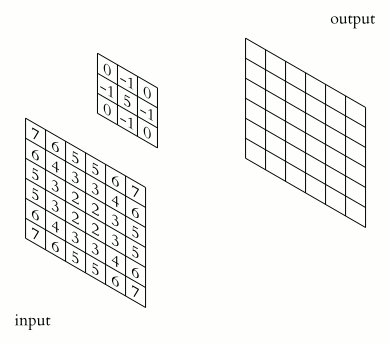pooling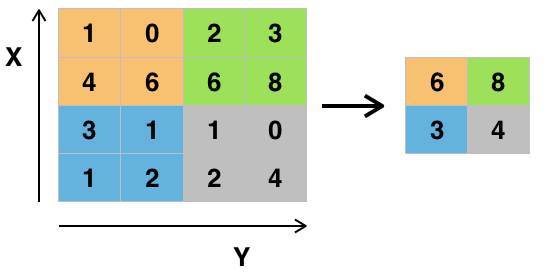$\qquad$ convolution $\ \to \$ feature map pooling : subsampling : dimensionality reduction : e.g. max-pooling

what makes it work

• kernels:
: reduced number of weith
: translational invariance
• pooling
: non-linearity
: dimensionality reduction
• training via backpropagation
: max-pooling weights $\ 0/1$
: kernels optimized for each layer
• ReLu neuron (rectified linear units)
: scale invariance
: computational efficiency
: error (back-) propatationconvolution net - illustration

• Visualizing and understanding convolutional networks,
M.D. Zeiler and R. Fergus,
European Conference on Computer Vision. Springer 2014.
• 1.3M/50k/100k training/validation/test data (parameters/hyper-parameters/performance)
$256 \times 256$ images (down-sampled, RVB mean zero)
10 sub-crops of size $224\times 254$ per image (center/corners/flip)
1000 label categories
• 5 convolution layers, 3 fully connected (4096 neurons)
a single pooling layer (first $\to$ second)
96 kernels of size $11 \times 11 \times 3$ (x,y, color); stride 4 (first layer)
256 kernels of size $5 \times 5 \times 48$ (second layer)
384 kernels of size $3 \times 3 \times 256$ (third layer)
384 kernels of size $3 \times 3 \times 192$ (forth layer)
256 kernels of size $3 \times 3 \times 192$ fifth layer)
• 60 million parameters; batch size 128; 70 epochs; 12 days, one GPU
• inversion deconvolution net
: map feature activites back to input pixels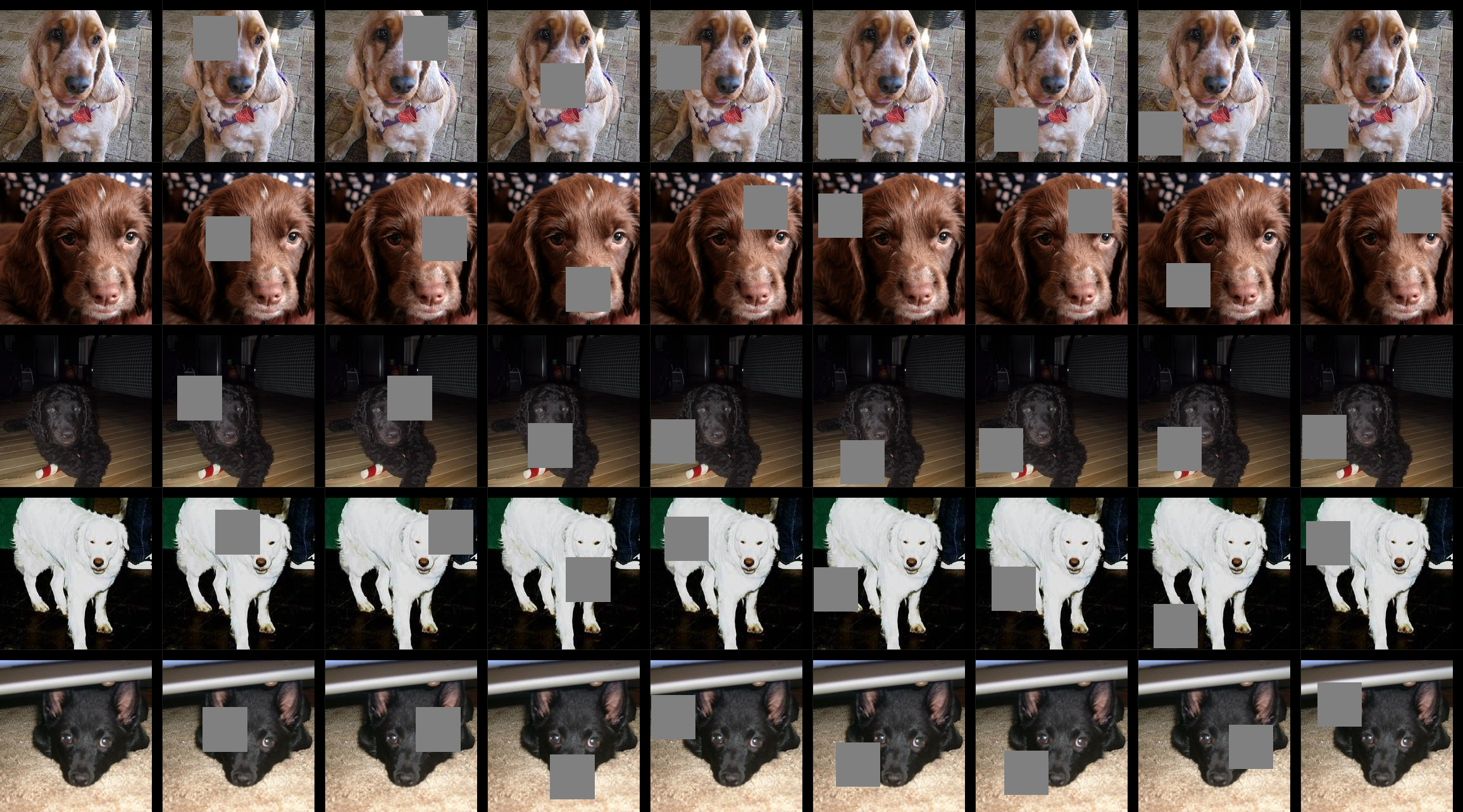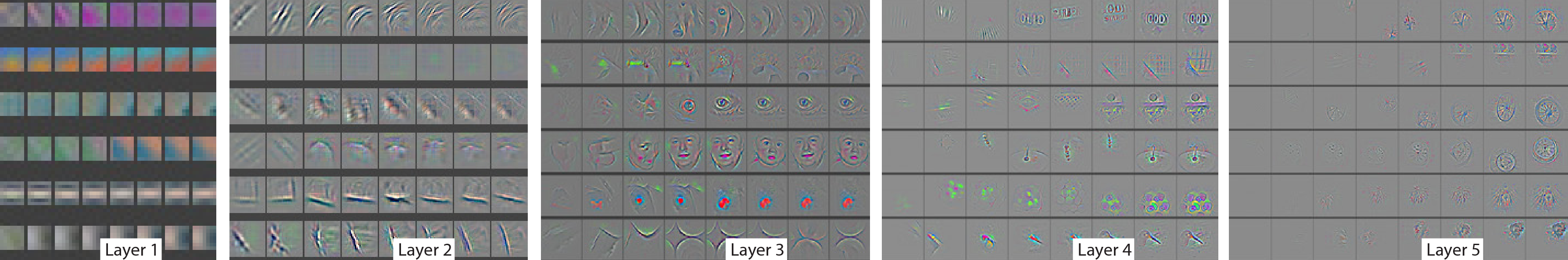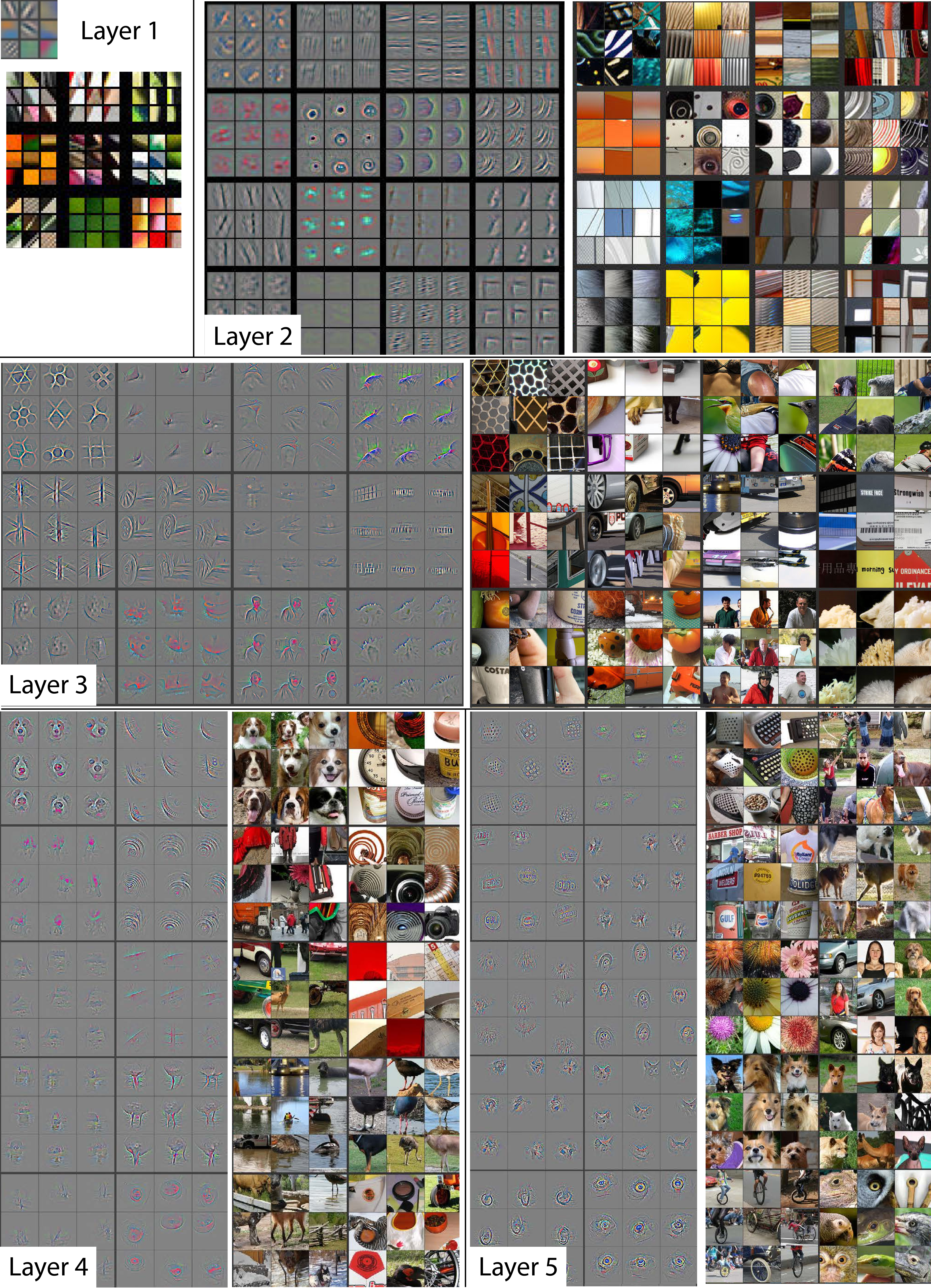fooling deep networks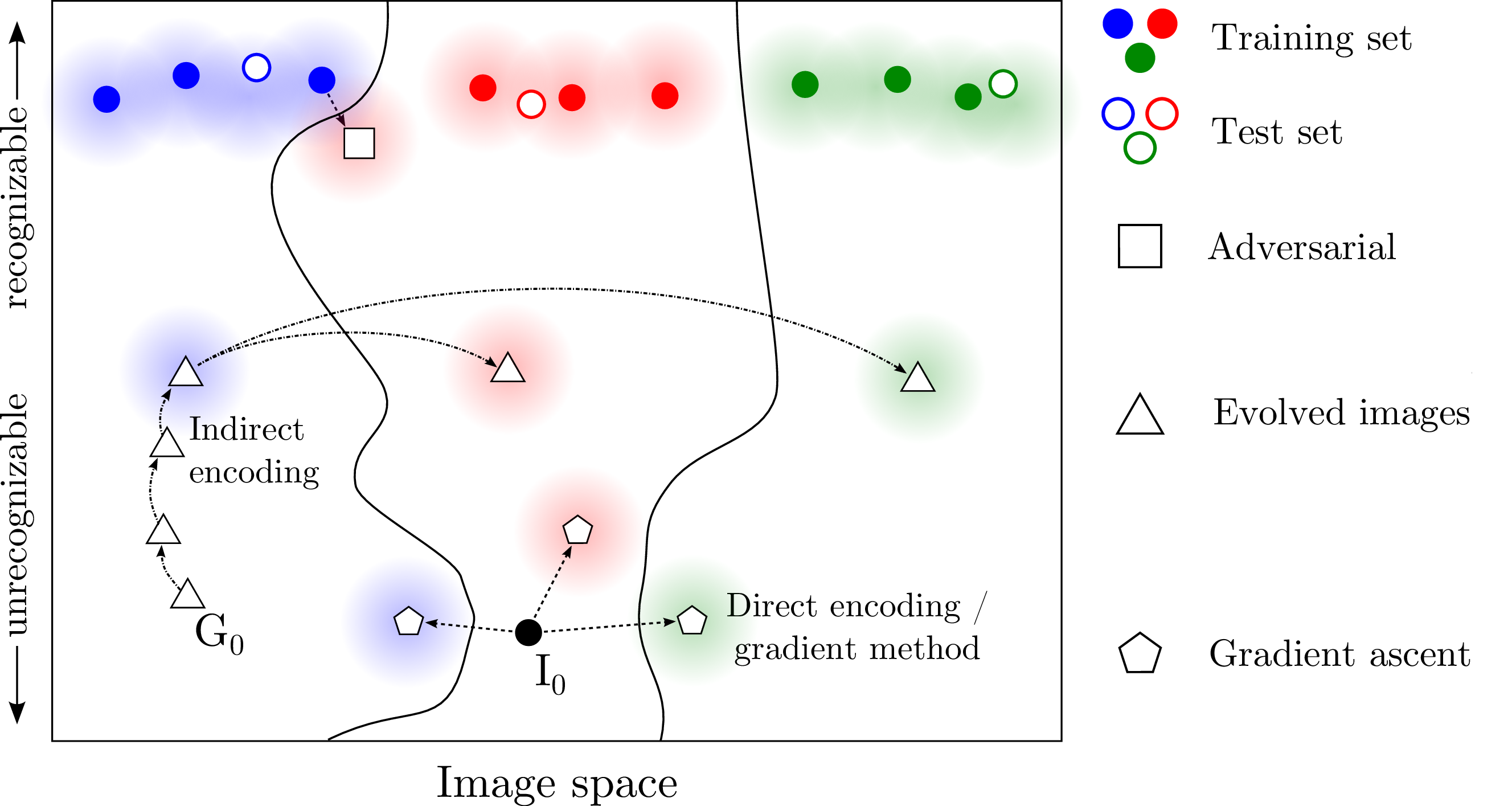• evolved recognizable images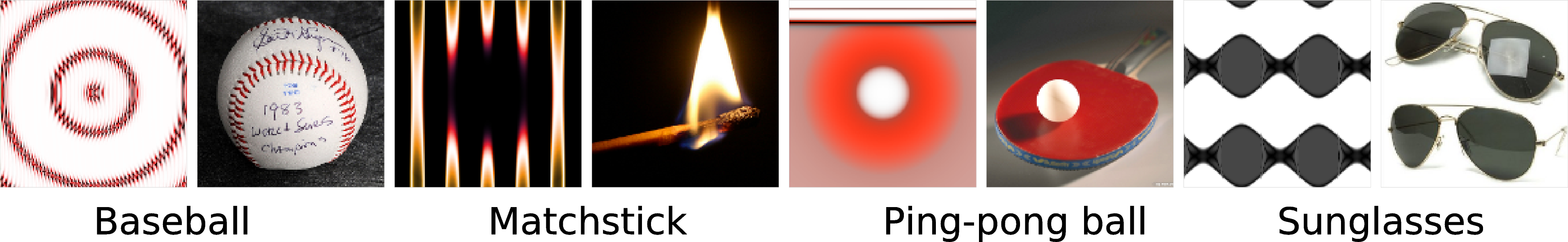• evolved unrecognizable images, mean confidence 99.12%
$\to \$ implications for "human-level performance"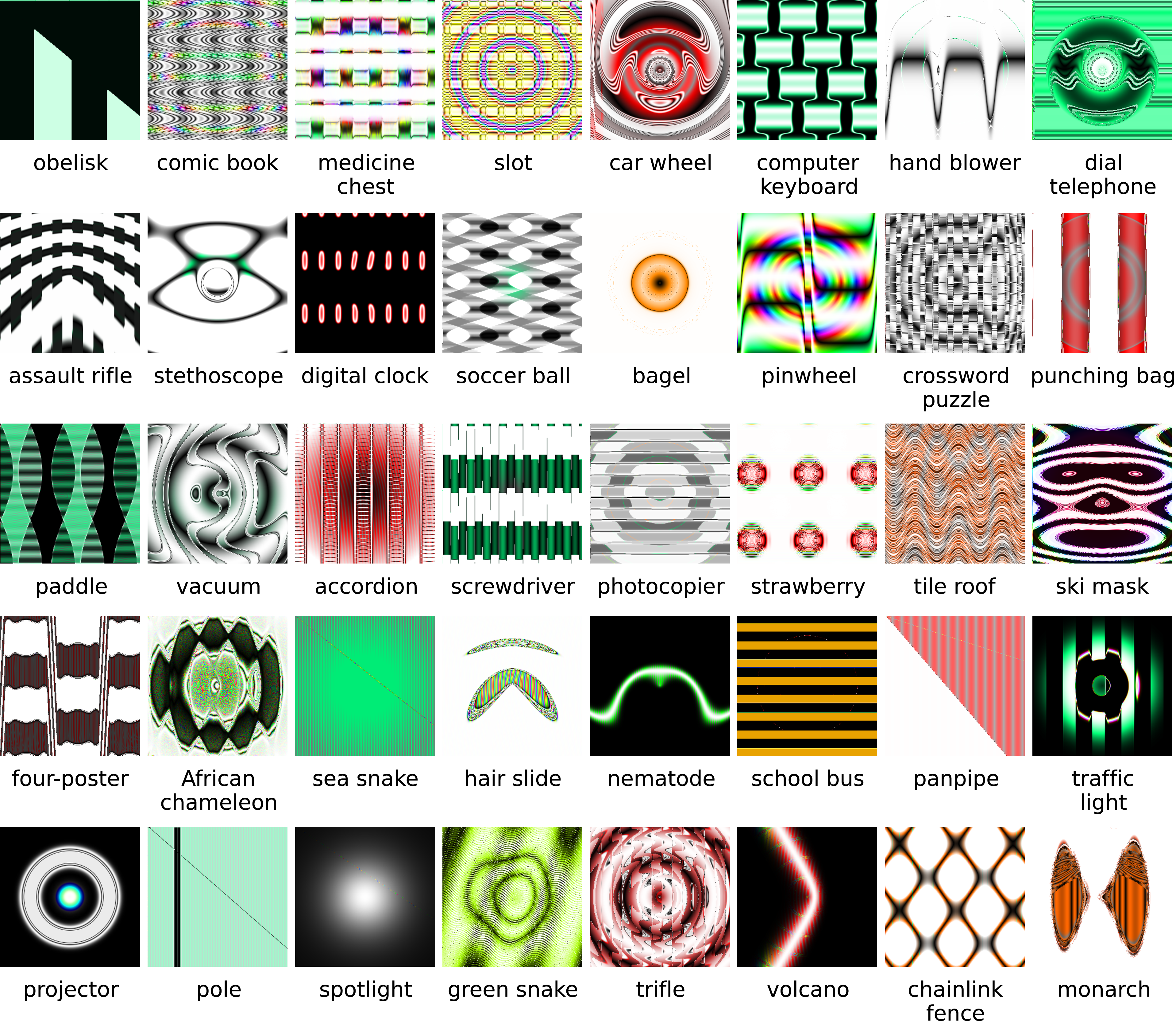+ 0.007 x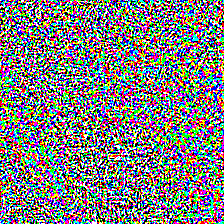=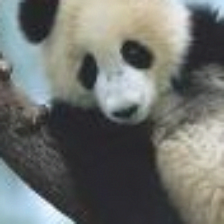"panda" "nematode" "gibbon" 57.7% confidence 8.2% confidence 99.3 % confidence

performance / confidance

• performante $\ \hat{=} \$ average success on test data
• confidence for a single prediction
: a range of methods
• most simple:
• diregard activities below a certain confidence threshold
• normalize activity of the remaining output neurons to unity
• confidence/class as the activity of most active output neuron

attacking deep networks

 original tempered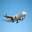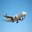image datasets

• CIFAR-10: 60000 32x32 color images in 10 different classes
• SVHN: Street View House Numbers (SVHN) Dataset

temper train data

• one random bit: RBB-blue set to zero
• training with tempered $\ \Leftrightarrow\$ testing with un-tempered data

missclassification induced by training data tempering

• percentage miss-classifaction
• selection of network architectures
$$\begin{array}{lcccc} \hline & \rlap{\text{baseline}} & & \rlap{\text{tampered}} \\ & \text{CIFAR} & \text{SVHN} & \text{CIFAR} & \text{SVHN} \\ \hline \text{optimal case} & 0 & 0 & 100 & 100 \\ \hline \text{BCNN} & 28.7 & 12.9 & 87.2 & 91.4 \\ \text{AlexNet} & 11.1 & 5.5 & 83.7 & 97 \\ \text{VGG-16} & 5.3 & 3.7 & 90.1 & 98.9 \\ \text{ResNet-18} & 23.8 & 3.6 & 42.4 & 40.9 \\ \text{SIRRN} & 4.7 & 3.9 & 74.1 & 89.5 \\ \text{DenseNet-121} & 2.6 & 2.6 & 60.7 & 68.1 \\ \hline \end{array}$$

TensorFlow

• TensorFlow is an open source machine learning framework (Google)
"state of the art deep learning for dummies"
• Phyton interface to Phyton/C++ code
• loop-free graphs
• edges: matrices of data (tensors, if higher-dimensional)
• vertices: machine-learning operations
• specific hardware (chips): Tensor Processing Unit (TPU)

import tensorflow as tf             // import tensorflow library as "tf"
mnist = tf.keras.datasets.mnist     // MNIST handwritten digits dataset already included

x_train, x_test = x_train / 255.0, x_test / 255.0

model = tf.keras.models.Sequential([                        // define your DL model
tf.keras.layers.Flatten(),
tf.keras.layers.Dense(512, activation=tf.nn.relu),        // use Relu neurons
tf.keras.layers.Dropout(0.2),
tf.keras.layers.Dense(10, activation=tf.nn.softmax)
])
loss='sparse_categorical_crossentropy',
metrics=['accuracy'])

model.fit(x_train, y_train, epochs=5)                       // trains model
model.evaluate(x_test, y_test)                              // tests and evaluates model

AlphaGo zerogame of Go

• number of configurations
$3^{19\cdot19} \approx 1.7\cdot10^{172}$
• 2017 AlphaGo zero (Goggle DeepMind)
: self-play ($5\cdot10^6$ games)
• Monte Carlo tree search + deep neural net
: estimating
• value function (of a new configuration)
• policy (likelihood to select an untested move)
• AlphaGo cheat sheet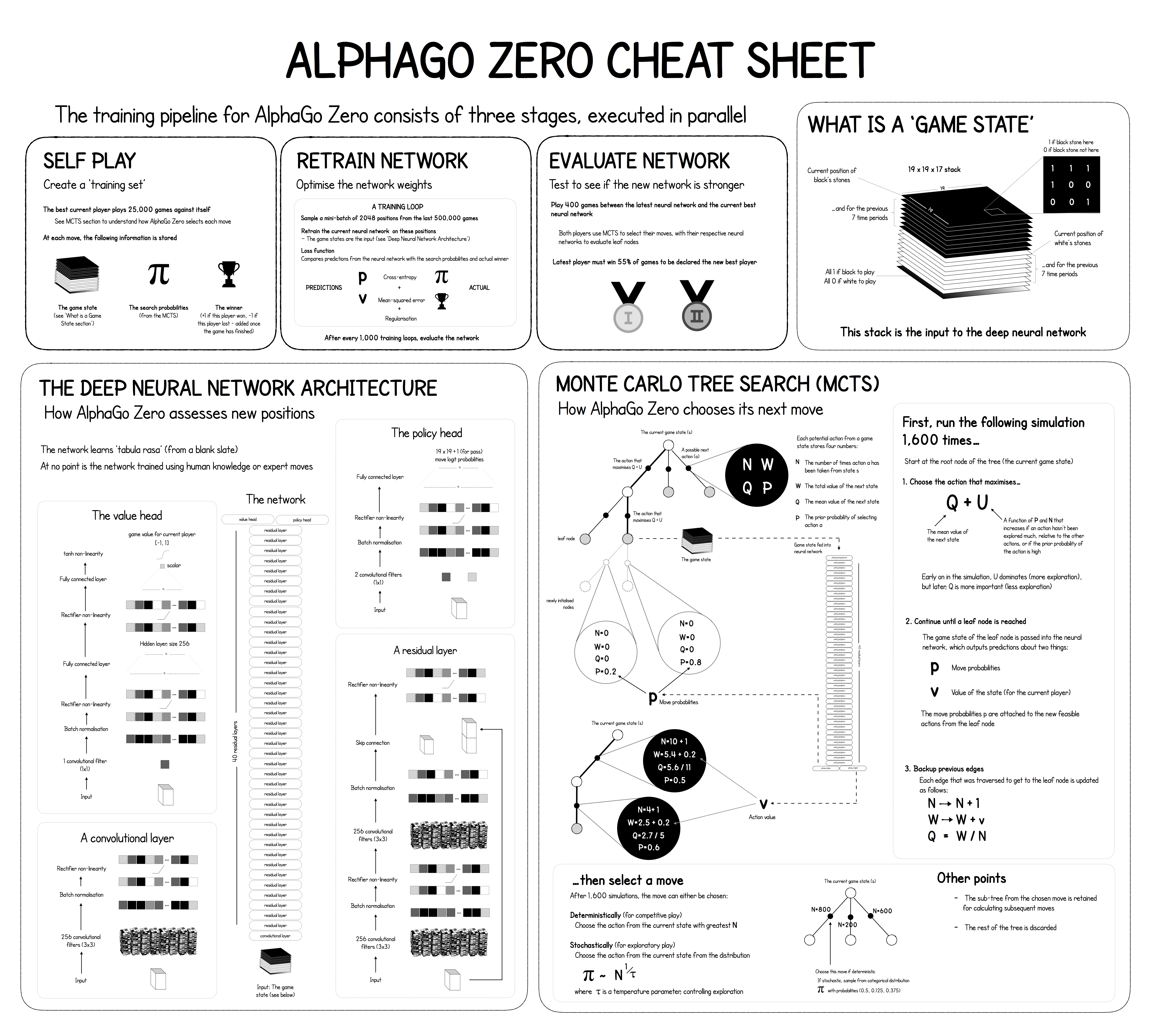a short history of the future

• univeral computing [Turing, 1936]
• artificial neurons [McCulloch and Pitts, 1943]
• Dartmouth conference 1956)
term "artificial intelligence" (AI) framed
McCarthy, Minsky, Shannon, ...
$\to \$ AI hype (human-level AIs within 10 years)
• perceptron [Rosenblatt, 1958]
• receptive fields in the brain [Hubel and Wiesel, 1959]
• single layer perceptron as linear classifiers [Minsky and Papert, 1969]
$\to \$ AI winter
• multi-layer perceptrons; back-propagation [Rumelhart et al, 1986]
$\to \$ ice slowly melting
• SVMs, Bayesian networks (1990s - ...)
• deep learning [Hinton et al, 2006]
$\to \$ revival
• successes in applications; conference presentations
• Acoustic Modeling using Deep Belief Networks
[Mohamed, Dahl and Hinton, 2010]
• Context-Dependent Pre-trained Deep Neural Networks
for Large Vocabulary Speech Recognition
[Dahl, Yu, Deng and Acero, 2012]
• ImageNet classification with deep convolutional neural networks
[Krizhevsky, Sutskever and Hinton, 2012]
$\to \$ second hype
• machine learing meta tools